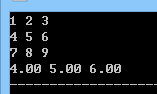2022-05-14 23:00

# 需要写个程序符合4条要求

``````a. 举例：

假设3行3列的矩阵为

1   2   3

4   5   6

7   8   9

则输出结果为4.00   5.00   6.00（保留两位小数）

b. 说明：

i. 程序中包含主函数main()和子函数，子函数的功能是对矩阵按列求均值

ii. 在主函数中定义二维数组和一维数组，二维数组中元素的赋值（从键盘输入）是在主函数中实现

iii. 将二维数组和一维数组作为子函数参数，参数传递方式为地址传递，在子函数中使用嵌套两重循环计算一维数组中每个元素的值；子函数的返回值为void类型

iv. 在主函数中输出一维数组中每个元素的值，保留两位小数
``````
• 写回答
• 好问题 提建议
• 追加酬金
• 关注问题
• 邀请回答

#### 2条回答默认 最新

•ByteSniper 2022-05-14 23:52
最佳回答
``````#include <stdio.h>
void f(double a,double ans)
{
int i = 0,j = 0;
for(i = 0; i < 3; ++i)
{
for(j = 0; j < 3; ++j)
{
ans[i] += a[j][i];
}
}
for(i = 0; i < 3; ++i) ans[i] /= 3;
}

int main()
{
double a;
double ans = {0};
int i = 0,j = 0;
for(i = 0; i < 3; ++i)
{
for(j = 0; j < 3; ++j)
{
scanf("%lf",&a[i][j]);
}
}
f(a,ans);
for(i = 0; i < 3; ++i) printf("%.2lf ",ans[i]);
return 0;
}
``````评论
解决 1 无用
打赏 举报 编辑记录